Methods and formulas for probabilities of missclassification in Nested Gage R&R Study

Select the method or formula of your choice.

Probabilities of misclassification

Minitab calculates the probabilities of misclassifications as both joint probabilities and conditional probabilities when you enter at least one specification limit.

Joint probabilities

Probability that part is bad and you accept it: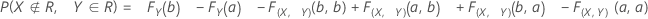Probability that part is good and you reject it: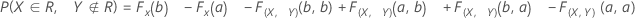Conditional probabilities

Probability that given a part is bad, you accept it (false accept):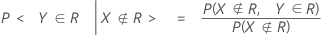Probability that given a part is good, you reject it (false reject):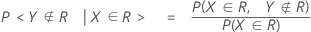Notation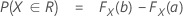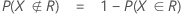F(X,Y) is the cumulative distribution function (CDF) of the bivariate normal random vector (X,Y)T with:

mean, μ = (θ,θ)T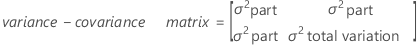F(X) and F(Y) are the corresponding marginal CDFs.

That is,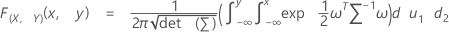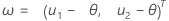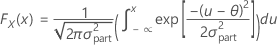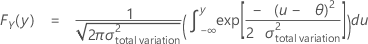By using this site you agree to the use of cookies for analytics and personalized content.  Read our policy